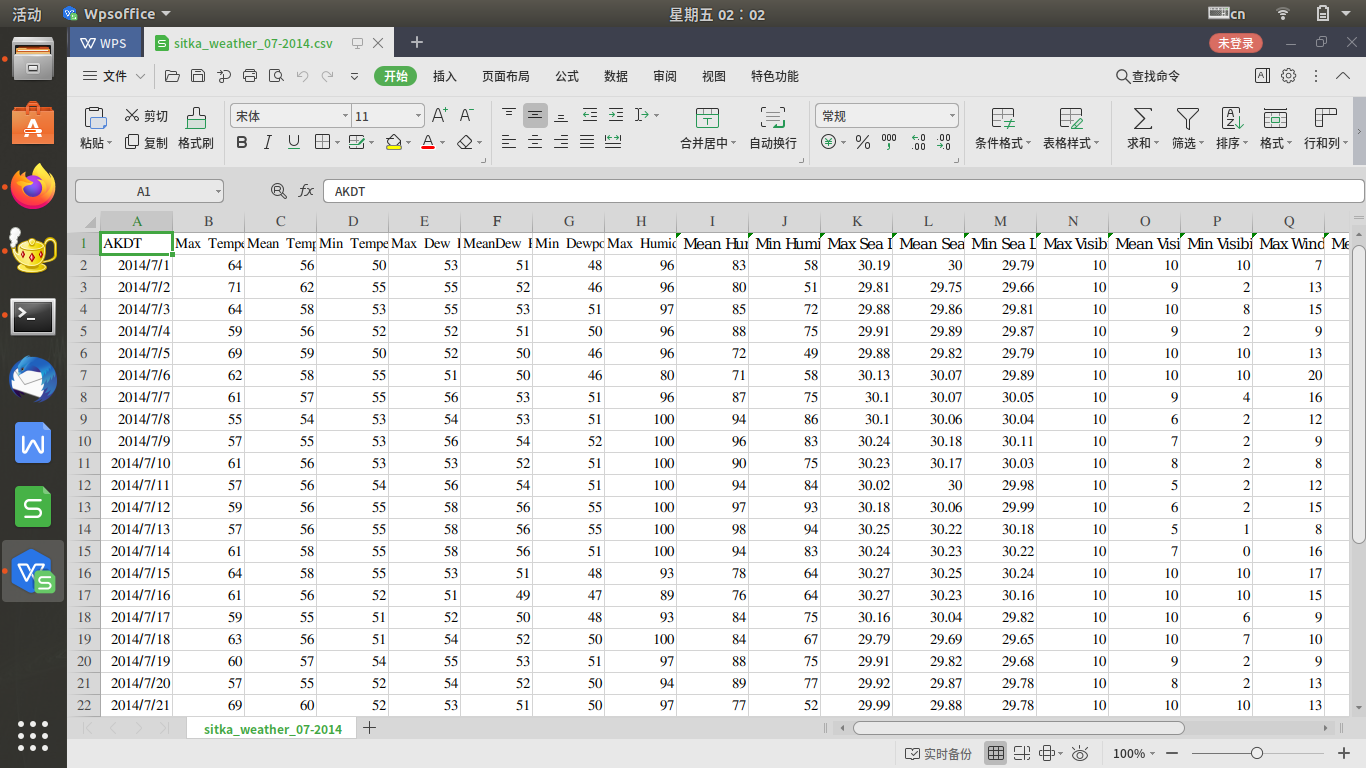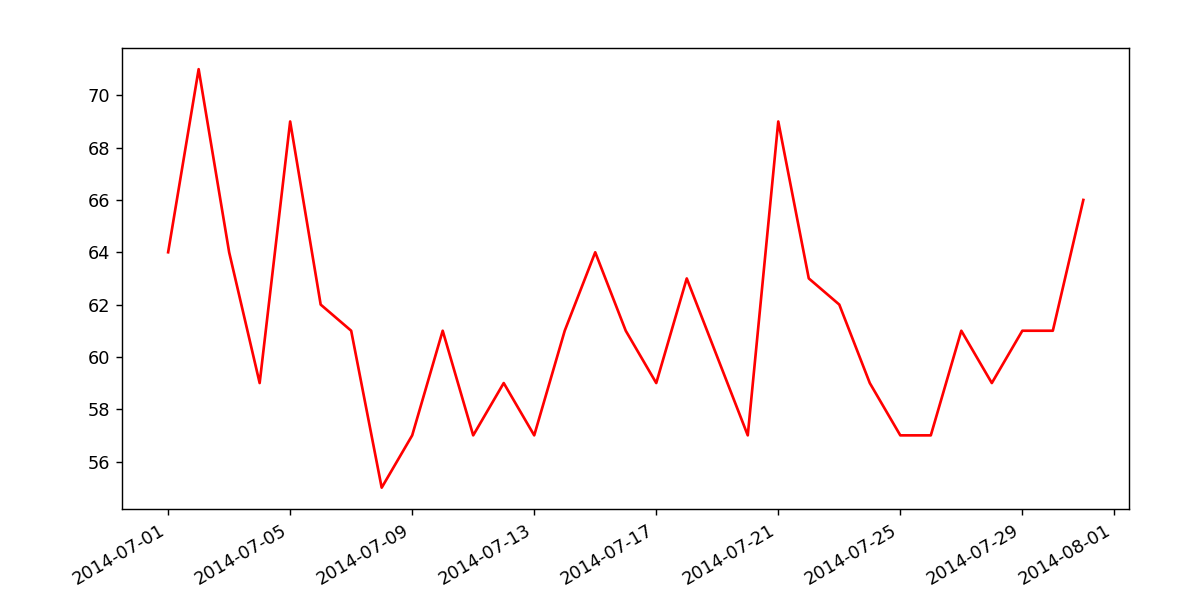1个回答

ax=fig.add_subplot(111)
ax.xaxis.set_major_locator(dates.DayLocator())matplotlib模块有问题，重装了也没用？

pycharm中matplotlib运行出现问题

<div class="post-text" itemprop="text"> <p>I have a python program that starts with: </p> <pre><code>from optparse import OptionParser import math #import wx import os import numpy as np import matplotlib.pyplot as plt from pylab import * from numpy import * import scipy as scipy from scipy import * from scipy import constants import scipy.signal as signal import matplotlib.pyplot as plt </code></pre> <p>It gives me error when I try to open it with php. I have googled and apparently if I do this before importing pylab or pyplot:</p> <pre><code>import matplotlib matplotlib.use('Agg') import matplotlib.pyplot as plt </code></pre> <p>Problem should be solved. But the error I get is:</p> <pre><code> /usr/lib/pymodules/python2.7/matplotlib/__init__.py:923: UserWarning: This call to matplotlib.use() has no effect because the the backend has already been chosen; matplotlib.use() must be called *before* pylab, matplotlib.pyplot, or matplotlib.backends is imported for the first time </code></pre> <p>Any idea what's going on??</p> </div>

win10中python的matplotlib库的相关问题

![如图所示，matplotlib按道理是安装成功的](https://img-ask.csdn.net/upload/201709/21/1506003881_125286.png) 但是运行代码的时候，出现了问题：  import matplotlib.pyplot as plt squares = [1,4,9,16,25] plt.plot(squares) plt.show()  ![图片说明](https://img-ask.csdn.net/upload/201709/21/1506003934_46507.png) 请问这该怎么解决？

python运行后显示matplotlib包有问题

 import matplotlib.pyplot as plt import numpy as np bar_width = 0.4 plt.bar(left = np.arange(len(school)),height = group_ms,label = 'MS', color = 'steelblue',width = bar_width) plt.bar(left = np.arange(len(school))+bar_width,height = group_gp,label = 'GP', color = 'indianred',width = bar_width) plt.xticks(np.arange(3)+0.2,school) plt.legend() plt.show()  错误代码是：  TypeError Traceback (most recent call last) <ipython-input-80-f63fd0192e45> in <module> 2 import numpy as np 3 bar_width = 0.4 ----> 4 plt.bar(left = np.arange(len(school)),height = group_ms,label = 'MS', color = 'steelblue',width = bar_width) 5 plt.bar(left = np.arange(len(school))+bar_width,height = group_gp,label = 'GP', color = 'indianred',width = bar_width) 6 plt.xticks(np.arange(3)+0.2,school) TypeError: bar() missing 1 required positional argument: 'x'  数据是 ![图片说明](https://img-ask.csdn.net/upload/201907/18/1563433306_197130.png) 需要将两个学校的数据进行对比

matplotlib共用x轴标签旋转问题

python matplotlib显示单色图问题

 def initArray(num): img = np.zeros((256,256)) for x in range(0,256): for y in range(0,256): img[x][y] = num return img fig = plt.figure() ax = fig.add_subplot() ax.imshow(initArray(20),cmap='gray') plt.show()  按说initArray的参数变化，显示的图片应该是不同的单色图，可是显示显示的全是黑色怎么回事？

Mac下的Anaconda 无法使用matplotlib.pyplot

File "/anaconda3/lib/python3.6/site-packages/matplotlib/__init__.py", line 891, in __getitem__ from matplotlib import pyplot as plt File "/anaconda3/lib/python3.6/site-packages/matplotlib/pyplot.py", line 32, in <module> import matplotlib.colorbar File "/anaconda3/lib/python3.6/site-packages/matplotlib/colorbar.py", line 28, in <module> import matplotlib.artist as martist AttributeError: module 'matplotlib' has no attribute 'artist'

python import matplotlib.pyplot 报错 DLL load failed

Anaconda3.5 python3.6 pycharm win7系统 import matplotlib没问题 matplotlib 2.1.0 import matplotlib.pyplot 报错 ![图片说明](https://img-ask.csdn.net/upload/201712/07/1512653783_182335.png) 另外，import matplotlib之前有个错误，更新了numpy之后好了。 在网上没找到类似的问题

Mac系统上import matplotlib.pyplot as plt出现下面问题

RuntimeError: Python is not installed as a framework. The Mac OS X backend will not be able to function correctly if Python is not installed as a framework. See the Python documentation for more information on installing Python as a framework on Mac OS X. Please either reinstall Python as a framework, or try one of the other backends. If you are using (Ana)Conda please install python.app and replace the use of 'python' with 'pythonw'. See 'Working with Matplotlib on OSX' in the Matplotlib FAQ for more information. matplotlib这个模块我是通过pycharm直接安装的，运行在venv里，没有装AnaConda

python用matplotlib画K线

import matplotlib.pyplot as plt import matplotlib.finance as mpf with open('SH#600004.txt') as obj: text=obj.readlines() baseinfo=text dayinfo=text[2:-1] date_list =[dayinfo[i].split(',') for i in range(len(dayinfo))] open_list =[dayinfo[i].split(',') for i in range(len(dayinfo))] high_list =[dayinfo[i].split(',') for i in range(len(dayinfo))] low_list =[dayinfo[i].split(',') for i in range(len(dayinfo))] close_list=[dayinfo[i].split(',') for i in range(len(dayinfo))] quotes=zip(date_list,open_list,high_list,low_list,close_list) #N=100 #open_list[:N],high_list[:N],low_list[:N],close_list[:N] fig,ax=plt.subplots() mpf.candlestick_ohlc(ax,quotes,width=0.6,colorup='r',colordown='green') plt.title(baseinfo) plt.xlabel(date_list) plt.rcParams['font.sans-serif']=['SimHei'] #用来正常显示中文标签 plt.rcParams['axes.unicode_minus']=False #用来正常显示负号 plt.show() **#以下是报错信息 C:\ProgramData\Anaconda3\python.exe G:/python/test/test.py C:\ProgramData\Anaconda3\lib\site-packages\matplotlib\cbook\deprecation.py:106: MatplotlibDeprecationWarning: The finance module has been deprecated in mpl 2.0 and will be removed in mpl 2.2. Please use the module mpl_finance instead. warnings.warn(message, mplDeprecation, stacklevel=1) Traceback (most recent call last): File "G:/python/test/test.py", line 18, in <module> mpf.candlestick_ohlc(ax,quotes,width=0.6,colorup='r',colordown='green') File "C:\ProgramData\Anaconda3\lib\site-packages\matplotlib\finance.py", line 737, in candlestick_ohlc alpha=alpha, ochl=False) File "C:\ProgramData\Anaconda3\lib\site-packages\matplotlib\finance.py", line 794, in _candlestick height = open - close TypeError: unsupported operand type(s) for -: 'str' and 'str'** 我搜了下知道日期格式转换需要用到date2num这个函数 我的文本里面日期格式是XXXX/XX/XX的形式 请问quotes一项里日期需求的格式是什么?

vs中使用python操作matplotlib时发生错误

win10下python中matplotlib相关问题

![如图显示matplotlib库安装完成](https://img-ask.csdn.net/upload/201709/21/1506002964_678793.png) ![但是在运行程序后出现这个问题](https://img-ask.csdn.net/upload/201709/21/1506002996_811603.png) 请问这是什么原因？该怎么解决？ 代码如下：  import matplotlib.pyplot as plt squares = [1,4,9,16,25] plt.plot(squares) plt.show() 

“亚马逊丛林里的蝴蝶扇动几下翅膀就可能引起两周后美国德州的一次飓风……” 这句人人皆知的话最初用来描述非线性系统中微小参数的变化所引起的系统极大变化。 而在更长的时间尺度内，我们所生活的这个世界就是这样一个异常复杂的非线性系统…… 水泥、穹顶、透视——关于时间与技艺的蝴蝶效应 公元前3000年，古埃及人将尼罗河中挖出的泥浆与纳特龙盐湖中的矿物盐混合，再掺入煅烧石灰石制成的石灰，由此得来了人...

C++11：一些微小的变化（新的数据类型、template表达式内的空格、nullptr、std::nullptr_t）

HashMap底层实现原理，红黑树，B+树，B树的结构原理 Spring的AOP和IOC是什么？它们常见的使用场景有哪些？Spring事务，事务的属性，传播行为，数据库隔离级别 Spring和SpringMVC，MyBatis以及SpringBoot的注解分别有哪些？SpringMVC的工作原理，SpringBoot框架的优点，MyBatis框架的优点 SpringCould组件有哪些，他们...

【阿里P6面经】二本，curd两年，疯狂复习，拿下阿里offer

《经典算法案例》01-08：如何使用质数设计扫雷（Minesweeper）游戏

《Oracle Java SE编程自学与面试指南》最佳学习路线图（2020最新版）